arrow_back_iosQuestions
help

# How to write formulas for the price?

check_circle
When creating discounts and surcharges, you specify the formula for calculating its amount. The system calculates it automatically when the order is placed. The amount must be negative for discounts and positive for surcharges. The formula can output an absolute value (in a currency) or a percentage of the cost. And most importantly, a formula can contain parameters and functions.

## Parameters

In the formula you can use the reservation parameters. The parameters should be enclosed in square brackets. For example: 100 * [PARAMETER].
To add parameters click the Insert parameter button.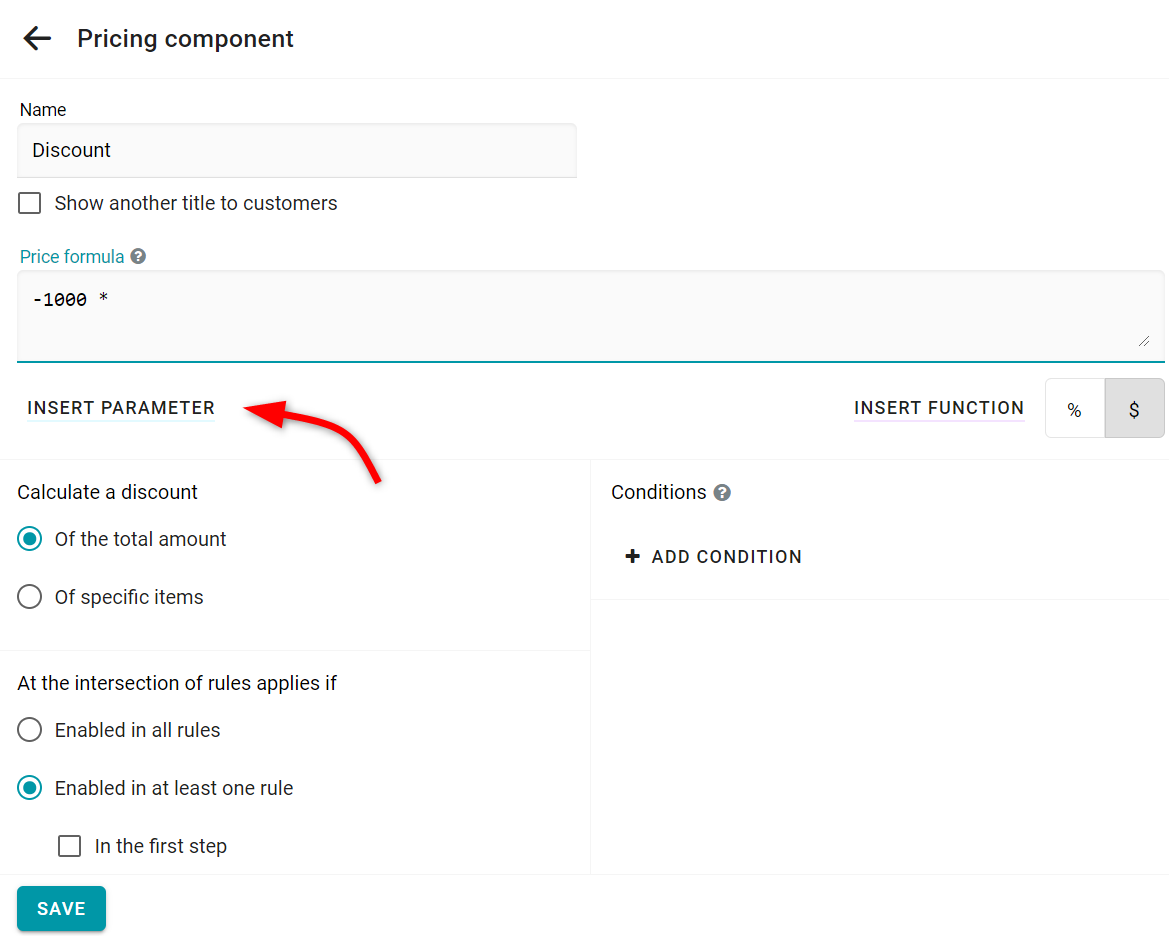Then pick a parameter you need.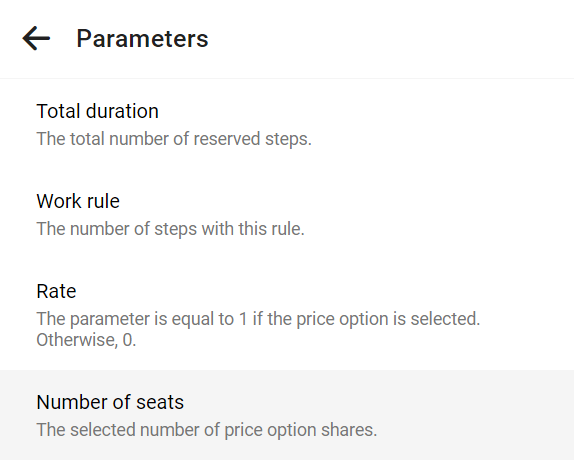The name for your parameter will be generated automatically. But you can change it if you want. After you click Insert the parameter will be inserted into the formula.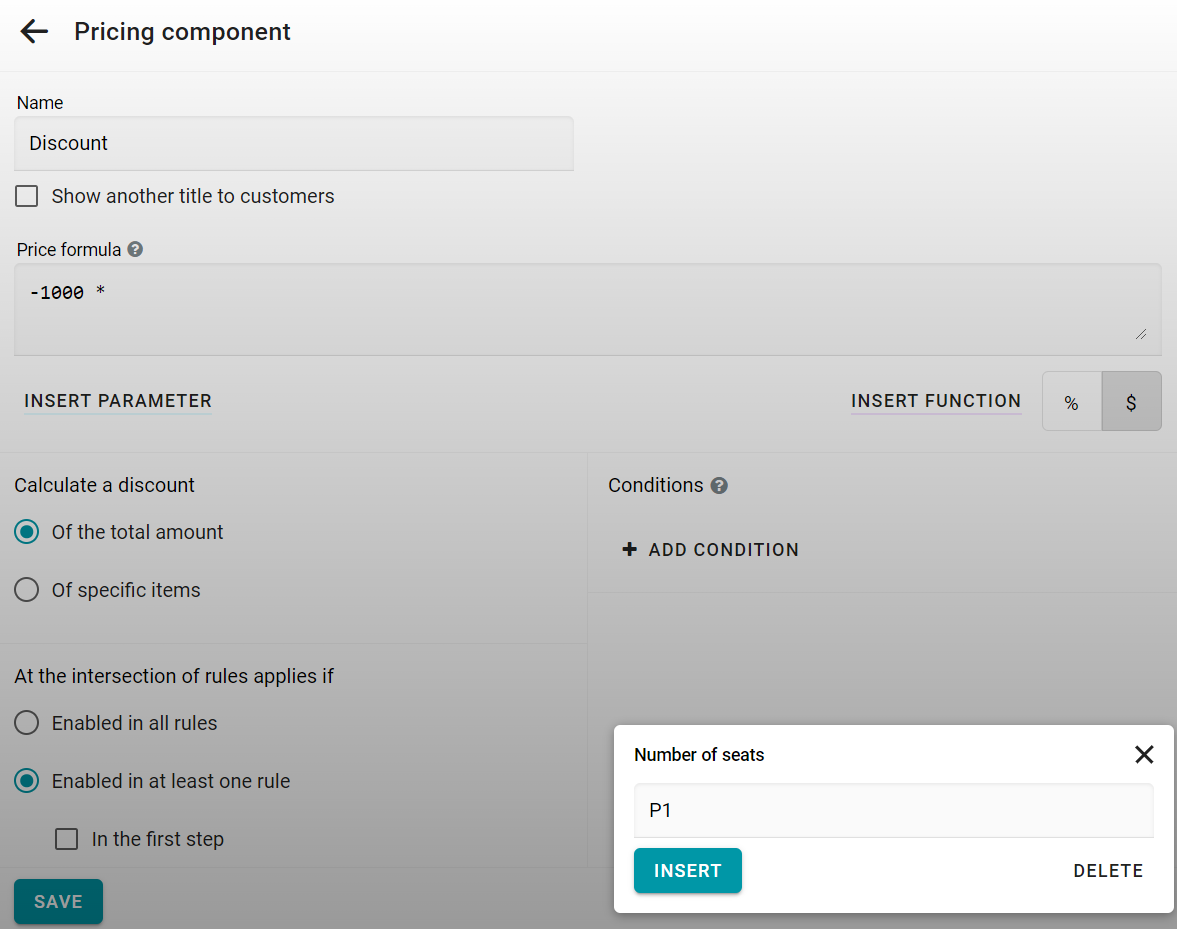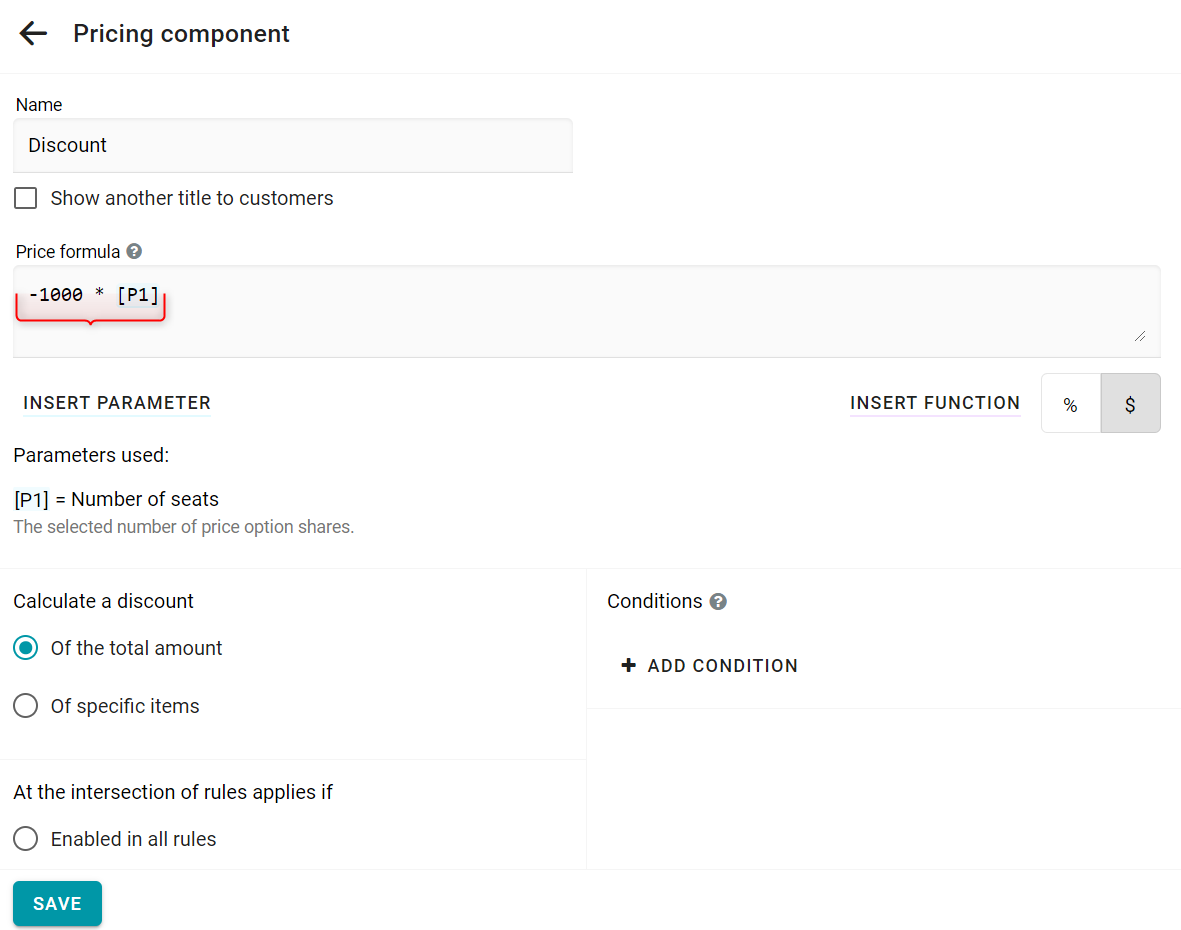The parameters of the formula are number of shares, not the price. For example, the number of tickets booked for a children's fare.
For price options for the whole resource, the parameter is set to 1 if the price option is reserved in the order. Otherwise, the parameter will be 0.
The Number field are passed to the formula as a number. The Checkbox field will be passed as 1 if it was set on the form. Otherwise, it is 0.
You can also use the [STEPS] parameter. It is equal to the number of reserved steps e.g. hours for hourly rent. Moreover, you can set such a parameter separately for each rule of work. These options are available only if the resource type is a rental.

## Functions

In addition to the usual arithmetic operations, you can use the following functions. To add a function click the Insert function button.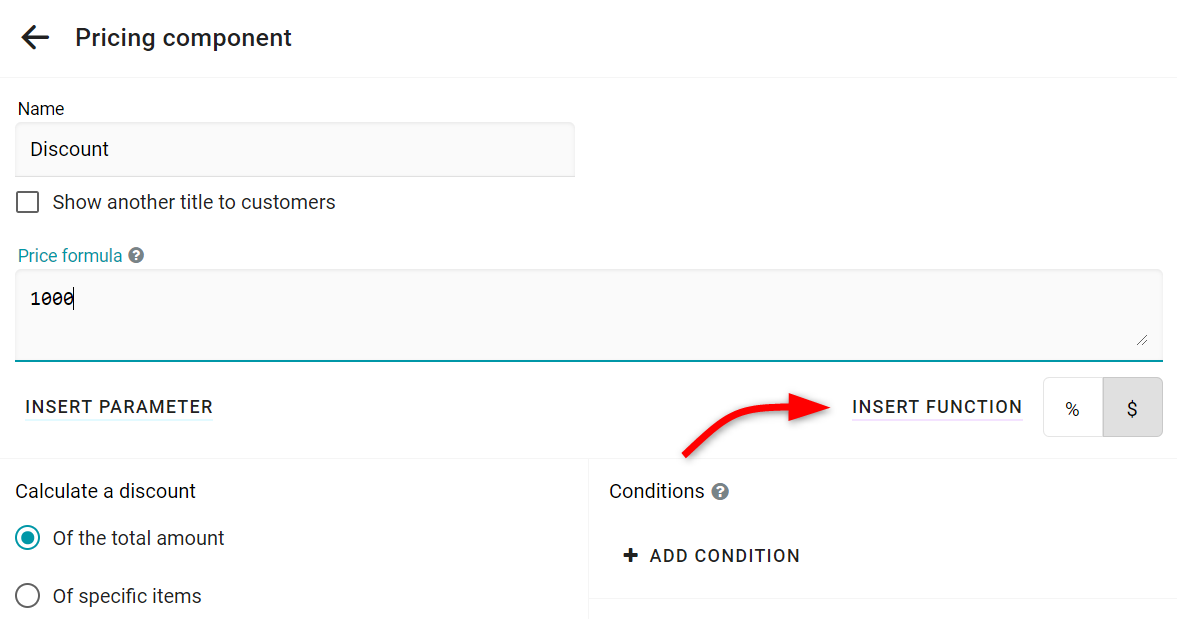Pick the necessary function from the list.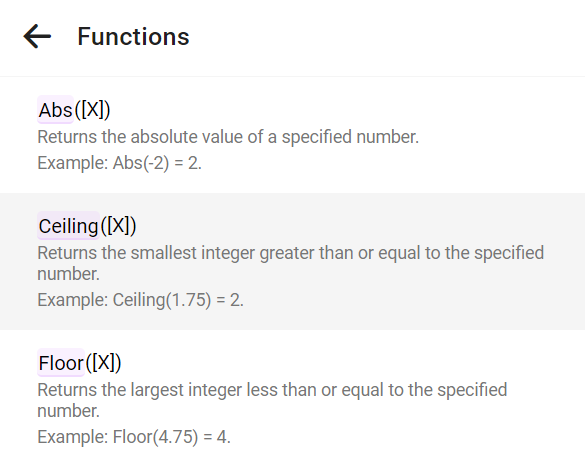The selected function will be inserted into the formula immediately.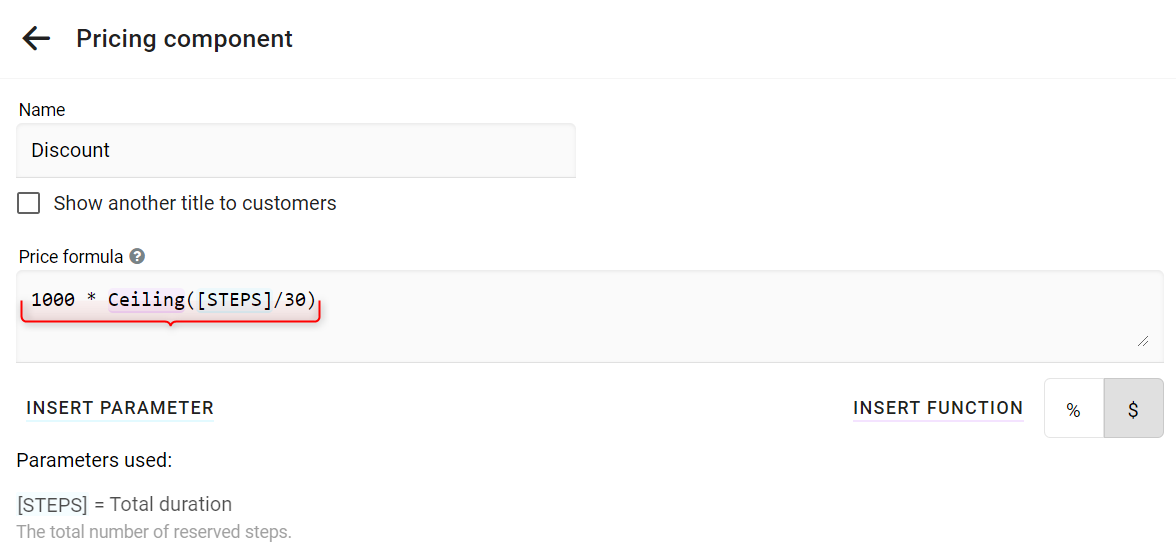The list of functions:
• Abs([X]) removes the minus of a negative number.
Example: Abs(-2) = 2.
• Ceiling([X]) rounds up to an integer.
Example: Ceiling(7.23) = 8.
• Floor([X]) rounds down to an integer.
Example: Floor(4.75) = 4.
• Max([X],[Y]) selects the greater of the two numbers.
Example: Max(7,8) = 8.
• Min([X],[Y]) selects the smaller of the two numbers.
Example: Min(7,8) = 7.
• Pow([X],2) calculates the first number in the power of the second.
Example: Pow(3,2) = 9.
• Round([X]) rounds the number to the nearest integer.
Example: Round(3.45) = 3.
• Round([X],2) rounds a number with precision.
Example: Round(3.4576,2) = 3.46.
• Sign([X]) for negative numbers returns -1. For positive 1.
Example: Sign(-99) = -1.
• Sqrt([X],2) calculates the square root.
Example: Sqrt(4) = 2.
• Truncate([X]) truncates the fractional part of a number.
Example: Truncate(15.7) = 15.
• if([CONDITION], [X], [Y]) computes the condition and returns [X], if it's true. Otherwise, it returns [Y]. In the condition, you can use these operations: >,>=,=,<,<=,<>, or, and.
Example: if([STEPS] > 5, 100, 200) = 100 for six reserved intervals.
• in([X], 1, 2, 3..) determines whether the number is in the sequence. The result can be used in the condition of the if function.
Example: in(7,1,7,8) = true.
The system also supports trigonometric and other functions: Sin([X]), Cos([X]), Tan([X]), Log([X],[Y]), Log10([X]), Exp([X]), Asin([X]), Acos([X]), Atan([X]), IEEERemainder([X],[Y]).